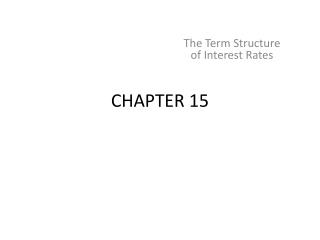Download PresentationCHAPTER 15

# CHAPTER 15 - PowerPoint PPT PresentationDownload Presentation## CHAPTER 15

- - - - - - - - - - - - - - - - - - - - - - - - - - - E N D - - - - - - - - - - - - - - - - - - - - - - - - - - -
##### Presentation Transcript

1. CHAPTER 15 The Term Structure of Interest Rates

2. Information on expected future short term rates can be implied from the yield curve The yield curve is a graph that displays the relationship between yield and maturity Three major theories are proposed to explain the observed yield curve Overview of Term Structure

3. Figure 15.1 Treasury Yield Curves

4. Bond Pricing • Yields on different maturity bonds are not all equal • Need to consider each bond cash flow as a stand-alone zero-coupon bond when valuing coupon bonds

5. Table 15.1 Yields and Prices to Maturities on Zero-Coupon Bonds (\$1,000 Face Value)

6. Using Spot Rates to Price Coupon Bonds • A coupon bond can be viewed as a series of zero coupon bonds. • To find the value each payment is discount at the zero coupon rate. • Once the bond value is found, one can solve for the yield. • It’s the reason that similar maturity and default risk bonds sell at different yields to maturity.

7. Sample Bonds A B Maturity 4 years 4 years Coupon Rate 6% 8% Par Value 1,000 1,000 Cash Flow in 1-3 60 80 Cash Flow in 4 1,060 1,080 Assuming Annual compounding

8. Price Using Spot Rates Bond A

9. Price Using Spot Rates Bond B

10. Solving For Yield to Maturity Bond A Bond Price 978.54 YTM 6.63% Bond B Price 1,047.56 YTM 6.61%

11. Yield Curve Under Certainty An upward sloping yield curve is evidence that short-term rates are going to be higher next year When next year’s short rate is greater than this year’s short rate, the average of the two rates is higher than today’s rate

12. Figure 15.2 Two 2-Year Investment Programs

13. Figure 15.3 Short Rates versus Spot Rates

14. Forward Rates from Observed Rates fn = one-year forward rate for period n yn = yield for a security with a maturity of n

15. Example 15.4 Forward Rates 4 yr = 8.00% 3yr = 7.00% fn = ? (1.08)4 = (1.07)3 (1+fn) (1.3605) / (1.2250) = (1+fn) fn = .1106 or 11.06%

16. Downward Sloping Spot Yield CurveExample Zero-Coupon RatesBond Maturity 12% 1 11.75% 2 11.25% 3 10.00% 4 9.25% 5

17. Forward Rates for Downward Sloping Y C Example 1yr Forward Rates 1yr [(1.1175)2 / 1.12] - 1 = 0.115006 2yrs [(1.1125)3 / (1.1175)2] - 1 = 0.102567 3yrs [(1.1)4 / (1.1125)3] - 1 = 0.063336 4yrs [(1.0925)5 / (1.1)4] - 1 = 0.063008

18. Interest Rate Uncertainty What can we say when future interest rates are not known today Suppose that today’s rate is 5% and the expected short rate for the following year is E(r2) = 6% then: The rate of return on the 2-year bond is risky for if next year’s interest rate turns out to be above expectations, the price will lower and vice versa

19. Interest Rate Uncertainty Continued Investors require a risk premium to hold a longer-term bond This liquidity premium compensates short-term investors for the uncertainty about future prices

20. Expectations Liquidity Preference Upward bias over expectations Theories of Term Structure

21. Expectations Theory Observed long-term rate is a function of today’s short-term rate and expected future short-term rates Long-term and short-term securities are perfect substitutes Forward rates that are calculated from the yield on long-term securities are market consensus expected future short-term rates

22. Long-term bonds are more risky Investors will demand a premium for the risk associated with long-term bonds The yield curve has an upward bias built into the long-term rates because of the risk premium Forward rates contain a liquidity premium and are not equal to expected future short-term rates Liquidity Premium Theory

23. Figure 15.4 Yield Curves

24. Interpreting the Term Structure • If the yield curve is to rise as one moves to longer maturities • A longer maturity results in the inclusion of a new forward rate that is higher than the average of the previously observed rates • Reason: • Higher expectations for forward rates or • Liquidity premium

25. Figure 15.5 Price Volatility of Long-Term Treasury Bonds

26. Figure 15.6 Term Spread: Yields on 10-Year Versus 90-Day Treasury Securities